CBSE (Science) Class 11CBSE
Share

# Plot the Corresponding Reference Circle for Each of the Following Simple Harmonic Motions. Indicate the Initial (T = 0) Position of the Particle, the Radius of the Circle, and the Angular Speed of the Rotating Particle. for Simplicity, the Sense of Rotation May Be Fixed to Be Anticlockwise in Every Case: (X Is in Cm And T Is In S) - CBSE (Science) Class 11 - Physics

ConceptVelocity and Acceleration in Simple Harmonic Motion

#### Question

Plot the corresponding reference circle for each of the following simple harmonic motions. Indicate the initial (= 0) position of the particle, the radius of the circle, and the angular speed of the rotating particle. For simplicity, the sense of rotation may be fixed to be anticlockwise in every case: (is in cm and is in s).

(a) = –2 sin (3+ π/3)

(b) = cos (π/6 – t)

(c) = 3 sin (2π+ π/4)

(d) = 2 cos πt

#### Solution 1

a)  x = -2 sin(3t + pi/3) = + 2 cos (3t + pi/3 + pi/2)

= 2 cos (3t + 5pi/6)

If this equation is compared with the standard SHM equation x = Acos (2pi/T t + phi) then we get:

Amplitude, A =  2cm

Phase angle, phi = (5pi)/6 = 150^@

Angular velocity, omega = 2pi/T =  3 "rad/sec"

The motion of the particle can be plotted as shown in the following figure.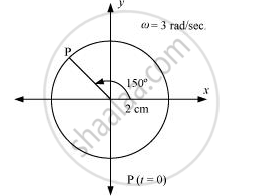If this equation is compared with the standard SHM equation x = A cos(2pi/T t + phi)

we get

Amplitude, A  = 1

Phase angle, phi = - pi/6 = - 30^@

Angular velocity, omega = (2pi)/T = 1 "rad/s"

The motion of the particle can be plotted as shown in the following figure.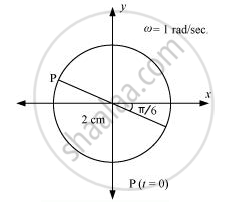c) x = 3 sin (2pit + pi/4)

= -3cos[(2pit + pi/4) + pi/2] = - 3 cos(2pit + (3pi)/4)

If this equation is compared with the standard SHM equation x = Acos((2pi)/T t + phi), then

we get:

Amplitude, A = 3 cm

Phase angle, phi = (3pi)/4 = 135^@

Angular velocity, omega = (2pi)/T = 2pi "rad/s"

The motion of the particle can be plotted as shown in the following figure.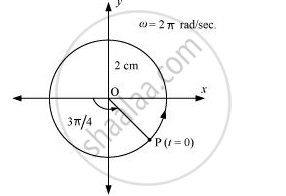d) x = 2 cos πt

If this equation is compared with the standard SHM equation c = Acos(2pi/T t  + phi)

#### Solution 2

a)  x = 2 cos (3t + pi/3 + pi/2)

Radius of the reference circle, r = amplitude of SHM = 2 cm

At  t = 0,  x = -2 sin pi/3 = (-2sqrt3)/2 =  -sqrt2 cm

Also omegat  =  3t :. omega  = 3 "rad/s"

cos phi_0 =  -sqrt(3)/2, phi_0 = 150^@

The reference circle is thus as plotted below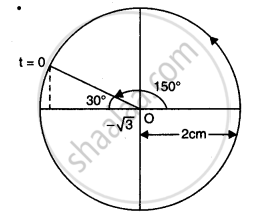b) x = cos (t -  pi/6 )

Radius of circle, r = amplitude of SHM = 1 cm

At t = 0, x = cos pi/6 =  sqrt3/2cm

cos phi_0 =  sqrt3/2, phi_0 = pi/6

The reference circle is thus as plotted below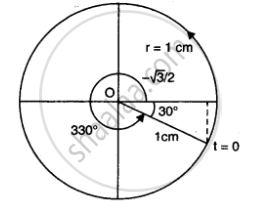c) x = 3 cos (2pit + pi/4 + pi/2)

Here , radius of reference circle, r = 3 cm and at t = 0, x = 3 sin pi/4  = sqrt3/2 cm

omegat = 2pit => omega = 2 pi "rad/s"

cos phi_0 = sqrt(3/2)/3 = 1/sqrt2

Therefore the reference circle is being show below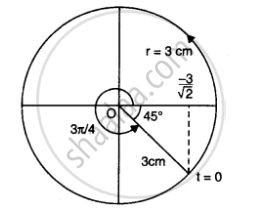d) x = 2 cos pit

Radius of reference circle, r = 2 cm and t = 0, x = 2cm

:. omegat = pit, or omega =  pi "rad/s"

cos phi_0 = 1, phi_0 = 0

The reference circle is plotted below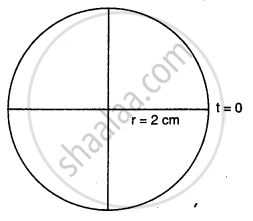Is there an error in this question or solution?

#### APPEARS IN

Solution Plot the Corresponding Reference Circle for Each of the Following Simple Harmonic Motions. Indicate the Initial (T = 0) Position of the Particle, the Radius of the Circle, and the Angular Speed of the Rotating Particle. for Simplicity, the Sense of Rotation May Be Fixed to Be Anticlockwise in Every Case: (X Is in Cm And T Is In S) Concept: Velocity and Acceleration in Simple Harmonic Motion.
S